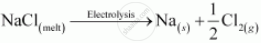# Name the processes from which chlorine is obtained as a by-product. What will happen if an aqueous solution of NaCl is subjected to electrolysis? - Chemistry

Name the processes from which chlorine is obtained as a by-product. What will happen if an aqueous solution of NaCl is subjected to electrolysis?

#### Solution

In the electrolysis of molten NaCl, Cl2 is obtained at the anode as a by product.

NaCl_(("melt")) -> Na_(  ("melt"))^+ + Cl_(    ("melt"))^(-)

At cathode: Na_(   ("melt"))^+ e^(-) -> Na_((s))

At anode : Cl_(  ("melt"))^+ -> Cl_((g)) + e^(-)

2Cl_((g)) -> Cl_(2(g))

The overall reaction is as follows:If an aqueous solution of NaCl is electrolyzed, Cl2 will be obtained at the anode but at the cathode, H2will be obtained (instead of Na). This is because the standard reduction potential of Na (E°= − 2.71 V) is more negative than that of H2O (E° = − 0.83 V). Hence, H2O will get preference to get reduced at the cathode and as a result, H2 is evolved.

NaCl_( (aq)) -> Na_( (aq))^+ + Cl_((aq))^-

At cathod: 2H_2O_((l)) + 2e^(-) -> H_(2(g)) + 2OH_((aq))^-

At anode : Cl_(("melt"))^(-) -> Cl_((g)) + e^(-)

2Cl_((g)) -> Cl_(2(g))

Concept: Oxidation Reduction
Is there an error in this question or solution?

#### APPEARS IN

NCERT Class 12 Chemistry
Chapter 6 General Principles and Processes of Isolation of Elements
Q 24 | Page 164